# Low complexity antenna selection for V-BLAST systems with OSIC detection

## Abstract

Multiple-input multiple-output (MIMO) systems have an advantage of spectral efficiency compared to single-input single-output systems, which means that the MIMO systems have significantly higher data throughput. The V-BLAST (Vertical Bell Laboratories Layered Space Time) scheme is a popular transceiver structure which has relatively good performance. In the V-BLAST scheme, ordered successive interference cancellation (OSIC) technique was proposed as a possible efficient detection method in terms of performance and complexity. However, MIMO systems suffer from high complexity and implementation cost. As a practical solution, a technique called antenna selection has been introduced. Since the existing literature considered only the capacity-based selection, we develop an optimal selection method for V-BLAST scheme using OSIC detection with respect to error rate performance in this article. Its complexity is shown to be proportional to the fourth power of the number of transmit antennas. To reduce the complexity without significant performance degradation compared to the optimal selection method, a near-optimal selection method is also proposed. Simulation results show that the proposed selection method is very close to the performance of optimal selection.

## Introduction

The spatial multiplexing systems with multiple transmit/receive antennas, referred to as multiple-input multiple-output (MIMO), have been developed to provide high data rate with limited bandwidth, i.e., high spectral efficiency. MIMO techniques can also be used to increase the diversity order for reliable transmission in a fading channel , . One of the implementation issues of MIMO systems is the increased hardware complexity and cost. A popular approach being employed to address the issue is the technique called the antenna selection. It has been shown that antenna selection maintains the same diversity order as the full antenna system, which makes antenna selection even more attractive (see , , and references therein).

In antenna selection, a critical issue is developing the selection method. In , those authors proposed selection methods based on minimum error rate for spatial multiplexing systems with linear and maximum likelihood (ML) receivers. Similar results based on the second-order statistic of the channel are also described in . Selection method when using the space-time coding was provided in . In , an optimal selection method for maximizing the capacity was proposed, and incremental or decremental selection methods as a greedy search algorithm were suggested to reduce the selection complexity in . For ML detection, the performance analysis of antenna selection was already studied in  by giving upper bounds on the pairwise error probability. However, the selection method for a nonlinear receiver such as successive interference cancellation (SIC) has not been investigated thoroughly. Even though antenna selection for V-BLAST (Vertical Bell Laboratories Layered Space Time) scheme was analyzed in , this article considered only the capacity as a performance metric as in the existing literature. In terms of error rate performance, few articles have considered antenna selection for the V-BLAST scheme using ordered successive interference cancellation (OSIC) detection since the detection order makes the analysis more complicated.

In this article, we focus on the antenna selection for V-BLAST scheme using OSIC detection with respect to error rate performance. Basically, SIC has two operations: nulling and cancellation. Common methods for the nulling step are the minimum mean square error (MMSE) and zero-forcing (ZF). In addition, it is well known that the detection order is critical to the overall performance. The optimal detection order is obtained by choosing a stream with the largest signal-to-noise and interference ratio (SINR) at each stage of the detection process as shown in .

Considering the optimal detection order, we derive an alternative expression for SINR using QR decomposition of which the derivation is similar to . The optimal antenna selection method will be derived using this expression. However, obtaining the optimal detection order needs so many pseudo-inverse matrix operations as the number of transmit antennas. Therefore, the selection operation itself becomes very complex. In order to reduce the selection complexity, we will propose a new detection order with which we select the antenna subset. This new detection order can be obtained by only one pseudo-inverse matrix operation, and thus it can be very effective in the MIMO systems with a large number of antennas. We will show that the reduction in the selection complexity of the new detection order does not degrade the overall performance significantly by analysis and simulations.

The rest of this article is organized as follows. Section II describes the system and the channel model. In Section III, we review the OSIC detection used in the conventional V-BLAST scheme and derive an alternative expression for SINR. In Section IV, we derive the optimal antenna selection method, and propose new near-optimal selection method. In Section V, we discuss the complexity of both methods. Section VI shows the simulation results. Finally, we provide our conclusion in Section VII.

## System and channel model

MIMO wireless systems with T transmit and R receiver antennas are considered in this study. The number of available radio frequency chains are N and M, respectively. According to the selection criterion, N out of T and M out of R transmit and receive antennas, respectively, are selected. The channel has flat fading which is slowly time varying. We assume that the receiver can track the channel state information (CSI) perfectly, but the transmitter does not know CSI. Therefore, antenna selection should be carried out on the receiver side by using current CSI. The selected antenna indices are then fed back to the transmitter. We also assume that this feedback path has negligible error and delay.

After antenna selection is applied, the discrete time for the MIMO system model can be described as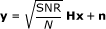(1)

where SNR is the expected signal-to-noise ratio at each receiver antenna, y represents the M × 1 received symbol vector, H is the M × N selected channel matrix, x is the N × 1 transmitted symbol vector, and n is the complex additive white Gaussian noise with variance 1/2 per each dimension. Each entry of the R × T original channel matrix is an i.i.d. circular symmetric complex Gaussian fading coefficient with zero mean and unit variance. The transmitted symbol is normalized such that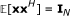, where I N is an N × N identity matrix. Throughout this article, (·) H and (·)denote the complex conjugate transpose and the transpose of a matrix, respectively. A (Moore-Penrose) pseudo-inverse of a matrix is represented by (·), and (·) i stands for the i th column of a matrix.

## OSIC detection algorithm

### A. ZF-based nulling

The OSIC with ZF as the nulling step had been described in . In order to derive an alternative expression for SINR at each detection stage, we summarize the overall process of ZF-OSIC detection algorithm as follows

initialization: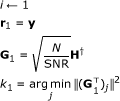recursion: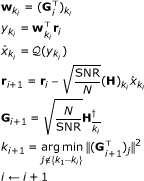where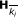denotes the matrix obtained by zeroing k1 to k i columns of H and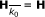. Assuming the previous detected symbol is correct and using the fact that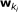vector is orthogonal to columns which is not yet detected and canceled, the post-detection SINR for the k i th stream is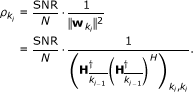(2)

We assume that the detection order is known in advance for the time being. This seems unreasonable at first, but from the procedure in , we can see that the detection order depends on only the channel matrix. In other words, the detection order is not affected by cancelation steps of the transmitted symbols. Therefore, if the current channel can be estimated at the receiver via training or pilot signals, then we can obtain the detection order for the antenna selection. Because antenna selection achieves larger gain in a slow-fading channel than in a fast-fading channel, the processing to obtain the detection order does not have to occur frequently. Thus, we can also use the detection order of the training signals.

Let the optimal detection order p = [k1, k2, ..., k N ]. Using this order, we can make the permutation matrix P as follows: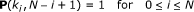(3)

where the size of P matrix is N × N, and k i is the integer number between 1 and N which is the i th element of p vector. To rearrange the columns of the channel according to the detection order, we multiply the channel matrix by the P matrix. It can easily be checked that this operation rearranges the columns of the channel matrix from the right to the left according to the detection order. The permutated channel matrix can be denoted by a QR decomposition.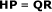(4)

where Q is an M × N matrix such that QHQ = I N , and R is an N × N upper triangular matrix. Now, using the definition of pseudo-inverse matrix H = (HHH)-1HH and P-1 = PH , we can get H = PR-1QH . Therefore, the following relation is obtained: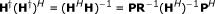(5)

Owing to the special structure of the P matrix, the diagonal element of denominator of (2), i.e., the norm of k i th row can be described as follows: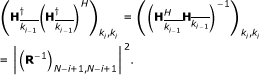(6)

Since (R-1) ii = 1/ R ii for an upper triangular matrix for all i, an alternative expression for the post-detection SINR of the k i th stream is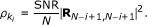(7)

That is, the SINR of each detection stage is proportional to the squared absolute value of diagonal elements of the R matrix. This result can be explained intuitively as follows. The pure permutation operation of the channel matrix does not affect the system performance. The detection order in HP is from the rightmost column to the leftmost column. In this case, the nulling vectoris the same as the conjugate of the (N - i + 1)th column of Q matrix, i.e.,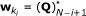. Therefore, the SINR at the first detection stage is proportional to the last diagonal element R N,N of R, the SINR at the second detection stage to RN-1 ,N-1, and so forth.

### B. MMSE-based nulling

In the case of the MMSE-based nulling, pseudo-inverse matrix operation should be changed as follows: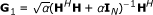(8)

where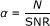. The ZF nulling completely eliminates the interference among transmitted streams at the expense of noise enhancement. On the other hand, the MMSE-based nulling pursues the balance of noise enhancement and estimation error. Here, we let the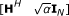be AH , then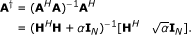(9)

Hence, G1 is the same as the first M columns of A.

Now, we can notice that the A has the pseudo-inverse form similar to the ZF equalizer, H. From this point of view, we can also express the alternative expression for SINR of MMSE-OSIC. In the same manner, we make the permutation matrix P under the assumption that we know the optimal detection order in advance. By considering the detection order, we make a modified augmented matrix: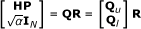(10)

where R is the N × N upper triangular matrix with real diagonal elements, and it is noted that the M × N upper matrix Q u may not be a unitary matrix. From the above equation, HP = Q u R.

The post-detection SINR of MMSE V-BLAST for the k i th stream is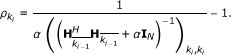(11)

Using the definition of pseudo-inverse, the following equality is established for the augmented matrix A: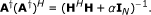(12)

By combining (10), (11), and (12) according to the similar argument of ZF-OSIC, the k i th post-detection SINR is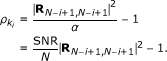(13)

The k i th SINR of MMSE-VBALST has the similar form as (7) except for the QR decomposition applied to the augmented matrix and the bias term of - 1.

## Selection criteria

Let us now develop the antenna selection method using the alternative expression for SINR discussed in the preceding section.

### A. Optimal selection methods

The error rate performance of a spatial multiplexing MIMO system is mostly affected by the stream with the smallest SINR. Therefore, it is optimal to choose the antenna subset maximizing the minimum value of diagonal elements of the R matrix for OSIC. The optimal selection criterion (SCopt) with respect to symbol error rate is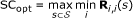(14)

where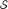is the set of all possible antenna combination, and s is the element set which consists of the selected antennas, 1 ≤ iN.

### B. Proposed selection method

The optimal method needs to compute a pseudo-inverse matrix operation at each iteration step to determine the detection order (p). In general, a pseudo-inverse of a matrix can be computed through singular value decomposition (SVD). As an alternative detection order, we propose to determine the detection order by a single pseudo-inverse as follows: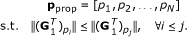(15)

That is, the detection order is determined by the row norms of G1 in the increasing order. To have some intuition, let us consider the ZF-OSIC algorithm again and assume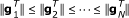, where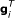stands for the i th row vector of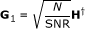. If the first stream is detected and canceled, then we have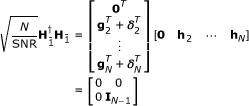(16)

where δ i represents the variation vector from G1. From this relation and HH = I N , we can induce following property for i = 2, 3, ..., N: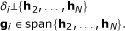(17)

That is, δ i is orthogonal to g i . Therefore each squared row norm of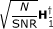is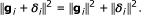(18)

However, we do not know the norm of variation vectors in advance. Therefore, it is possible to have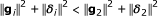for some i > 2. From this point on, the new detection order begins to deviate from the optimal order. If the variation vector has a small norm, then the new detection order will be nearly the same as the optimal detection order. Thus, we propose to use this new detection order for selecting the antenna subset. The P matrix can be produced through only one pseudo-inverse of the channel matrix H.

Since (17) is not satisfied for MMSE-OSIC, additional term begins to affect on the row norm as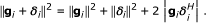(19)

Therefore, when the selection using new detection order is used for MMSE-OSIC, it is obvious that the performance gap from optimal selection case will be larger than in the ZF-OSIC case, which will be shown in simulation results. Of course, this analysis does not show the effect on the diagonal elements of the R matrix directly. However, we can conjecture that the variation vector has small norm for a random complex matrix. Therefore, the new detection order will be almost the same as the optimal order at least for the first few indices. Here, we notice that the first order is always the same for the optimal and the suboptimal order, but the tractable analysis on the overall effect of the detection order may be difficult as the number of transmit and receiver antenna increases.

## Complexity comparison

In this section, we evaluate the selection complexity quantitatively. One way to quantify this is with the notion of a flop which is a floating point operation. The complexity is measured by the number of flops, denoted as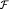. For an M × N matrix H M×N, a Classical Gram-Schmidt (CGS) algorithms for QR decomposition needs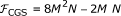flop operations . The flop count for SVD of a real valued M × N matrix is given by 4M2N +8MN2 +9N3 in Golub-Reinsch algorithm . The multiplication and the addition between two complex scalar values require six flops and two flops, respectively. Further, most operations in SVD are matrix multiplications, which in turn consist of several vector dot products. Each dot product of two real vectors with length N has the flop count of 2N, whereas the flop count for two complex vectors is 8N. That is, the SVD complexity of a complex matrix is four times higher than that of a real matrix approximately due to some additional scalar multiplications and additions. This is more accurate than  where the flop count of a complex SVD is approximated by six times that of a real SVD by treating every operation as complex multiplication. Thus, the flop count for SVD of a complex valued M × N matrix is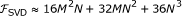.

To get the optimal detection order, we need N pseudo-inverse operations for the matrices where the size decreases from M × N to M × 1. Therefore, the total flop count for optimal order can be calculated by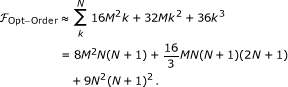(20)

Therefore, the total flop count of optimal selection is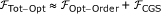(21)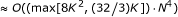(22)

where we set K = M/N as the ratio of the number of receiver antenna to that of transmit antenna, which is larger than one for a V-BLAST system. While the new detection order needs only one pseudo-inverse matrix operation, the total flop count of the proposed selection method is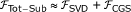(23)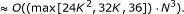(24)

Approximately, the proposed algorithm has 1/N times the complexity of the optimal selection method in flops for the case of K ≈ 1. Thus, the larger the number of transmit antenna N, the more the complexity reduction that we get for the proposed selection method.

## Simulation results

Monte Carlo simulations are performed for a wireless system with multiple antennas to evaluate the performance of the proposed selection criterion. For the sake of simplicity, we consider only transmit antenna selection. However, this simplification does not change the relative performance tendency for each criterion. Performance is measured based on vector symbol error ratio (VSER), which is obtained by averaging over 10,000 randomly generated channels according to i.i.d. distribution. We used QPSK modulation in all the simulations, and one frame consists of 100 vector symbols. In all the figures, two other methods are also compared. One is the well-known maximum capacity-based selection where a general capacity formula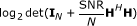is used. Implementing an algorithm to compute the determinant has the complexity order of O(N3). Compared to (24), the maximum capacity method has the same complexity order assuming that K is fixed, but the proposed method has better performance than the maximum capacity method in terms of VSER. The other is the non-selection case which means a system with fixed Tx and Rx antennas. For a fair comparison, the channel matrix of the non-selection case the size of which is the same as the selected channel matrix is generated according to i.i.d. circular symmetric complex Gaussian fading with zero mean and unit variance. It is noted that this case has no diversity advantage.

Figures 1 and 2 show the performance comparison of selection methods from a 3 × 4 system to a 3 × 3 system for ZF-OSIC and MMSE-OSIC where M × N denotes the size of channel matrix. It is shown that the optimal and the proposed methods have almost the same performance. At 10-3 VSER, the proposed algorithm gains about 1 dB compared to the maximum capacity-based selection method. That is, simple capacity-based selection does not work well in the MIMO system with nonlinear receiver using OSIC detection in terms of error rate performance. The antenna selection scheme using our proposed selection method has also the advantage of increased diversity.

In Figures 3 and 4, the performance of MIMO systems with larger number of antennas are compared. Almost the same relative performance tendency is obtained. The performance of the ZF-OSIC receiver shows still near-optimal performance in the case of the proposed method. On the other hand, the proposed method has small performance loss compared to the optimal case in MMSE-OSIC detection as shown in Figure 4. When the number of antennas increases, it is expected that the performance gap will grow larger, but the complexity reduction will be more significant.

## Conclusion

In this article, we have presented an alternative expression for SINR at each nulling and cancelation step using QR decomposition of a channel matrix. By means of this expression, we derive the optimal antenna selection method in a spatial multiplexing MIMO system using OSIC detection. To reduce the complexity of the selection process itself, we propose a low complexity selection method using a new detection order. The proposed order can be obtained by a single computation of pseudo-inverse of the channel matrix. Therefore, the selection complexity is reduced by a factor of about 1/N compared with the optimal selection complexity. Based on the simulations, we have shown that the proposed method has near-optimal performance for the MIMO systems with a small number of antennas. In the systems with a large number of antennas, we can achieve even higher complexity reduction without significant performance loss. The proposed selection method depends only on the current CSI, and thus, it can be applied to the systems with any quadrature amplitude modulation.

## Abbreviations

CGS:

Classical Gram-Schmidt

CSI:

channel state information

MIMO:

multiple-input multiple-output

MMSE:

minimum mean square error

OSIC:

ordered successive interference cancellation

SINR:

signal-to-noise and interference ratio

SVD:

singular value decomposition

V-BLAST:

Vertical Bell Laboratories Layered Space Time

VSER:

vector symbol error ratio

ZF:

zero-forcing.

## References

1. Foschini GJ, Gans MJ: On limits of wireless communications in a fading environment when using multiple antennas. Wirel Pers Commun 1998, 6(3):311-335. 10.1023/A:1008889222784

2. Telatar IE: Capacity of multi-antenna Gaussian channels. Eur Trans Telecommun 1999, 10(6):586-595.

3. Molisch AF, Win MZ: MIMO systems with antenna selection. IEEE Microwave 2004, 5(1):46-56. 10.1109/MMW.2004.1284943

4. Sanayei S, Nosratinia A: Antenna selection in MIMO systems. IEEE Commun Mag 2004, 42(10):68-73. 10.1109/MCOM.2004.1341263

5. Heath R, Paulraj A: Antenna selection for spatial multiplexing systems based on minimum error rate. Proc. IEEE International Conference on Communications Systems (ICC'01), Helsinki, June 11-14 2002, 7: 2276-2280.

6. Gore D, Heath R, Paulraj A: Statistical antenna selection for spatial multiplexing systems. Proc. IEEE International Conference on Communications Systems (ICC'02), New York, April 28-May 2 2002, 1: 450-454.

7. Gore D, Paulraj A: MIMO antenna subset selection with space-time coding. IEEE Trans. Signal Process 2002, 50(10):2580-2588. 10.1109/TSP.2002.803337

8. Blum RS, Winters JH: On optimum MIMO with antenna selection. IEEE Commun Lett 2002, 6(8):322-324. 10.1109/LCOMM.2002.802050

9. Gorokhov A, Gore D: A Paulraj, Receive antenna selection for MIMO flat-fading channels: theory and algorithm. IEEE Trans Inf Theory 2003, 49(10):2687-2696. 10.1109/TIT.2003.817458

10. Bahceci I, Duman TM, Altunbasak Y: Antenna selection for multiple-antenna transmission systems: performance analysis and code construction. IEEE Trans Inf Theory 2003, 49(10):2669-2681. 10.1109/TIT.2003.817455

11. Lu D, So DKC: Performance based receive antenna selection for V-BLAST systems. IEEE Trans Wirel Commun 2009, 8(1):214-225.

12. Golden GD, Foschini CJ, Valenzuela RA, Wolniansky PW: Detection algorithm and initial laboratory results using VBLAST space-time communication architecture. Electron Lett 1999, 35(1):14-16. 10.1049/el:19990058

13. Hassibi B: A fast square-root implementation for BLAST. Proceedings of the Thirty-Fourth Asilomar Conference on Signals, Systems and Computers, October 29-November 1 2000, 1255-1259.

14. Shen Z, Chen R, Andrews JG, Heath RW, Evans BL: Low complexity user selection algorithms for multiuser MIMO systems with block diagonalization. IEEE Trans Signal Process 2006, 35(1):3658-3663.

15. Golub GH, Loan CFV: Matrix Computations. 3rd edition. Johns Hopkins University Press, Baltimore, MD; 1983.

## Acknowledgements

This research was supported in part by the Basic Science Research Program (2010-0013397) and the Mid-career Research Program (2010-0027155) through the NRF funded by the MEST, Seoul R&BD Program (JP091007, 0423-20090051), the INMAC, and BK21.

## Author information

Authors

### Corresponding author

Correspondence to Jungwoo Lee.

### Competing interests

The authors declare that they have no competing interests.

## Authors’ original submitted files for images

Below are the links to the authors’ original submitted files for images.

## Rights and permissions

Reprints and Permissions

Bae, Y., Lee, J. Low complexity antenna selection for V-BLAST systems with OSIC detection. J Wireless Com Network 2011, 6 (2011). https://doi.org/10.1186/1687-1499-2011-6

• Accepted:

• Published:

• DOI: https://doi.org/10.1186/1687-1499-2011-6

### Keywords

• MIMO systems
• antenna selection
• V-BLAST
• QR decomposition
• OSIC detection
• low complexity